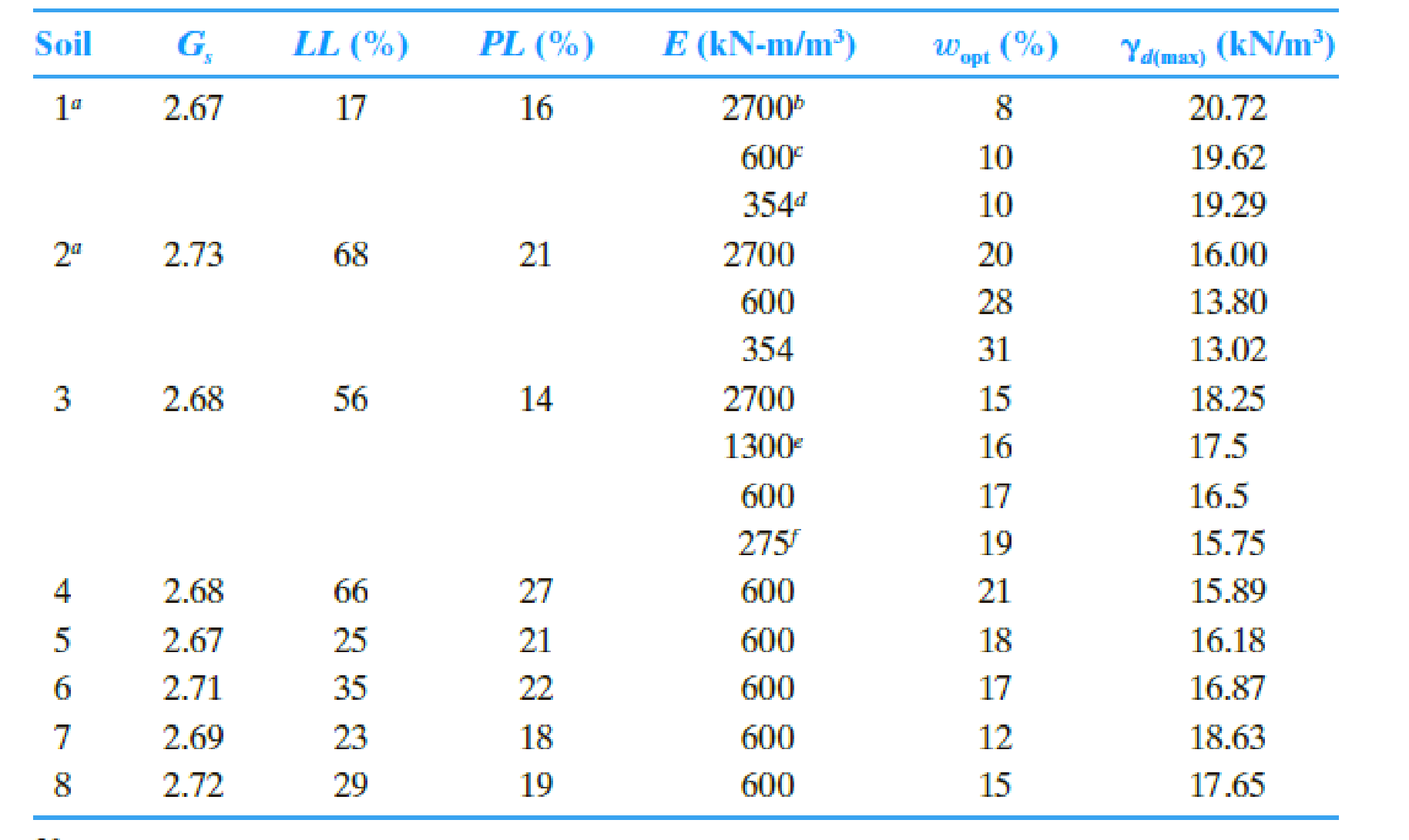Chapter 6, Problem 6.1CTPPrinciples of Geotechnical Enginee...

9th Edition
Braja M. Das + 1 other
ISBN: 9781305970939

Solutions

Chapter
SectionPrinciples of Geotechnical Enginee...

9th Edition
Braja M. Das + 1 other
ISBN: 9781305970939
Textbook Problem

Since laboratory or field experiments are generally expensive and time consuming, geotechnical engineers often have to rely on empirical relationships to predict design parameters. Section 6.6 presents such relationships for predicting optimum moisture content and maximum dry unit weight. Let us use some of these equations and compare our results with known experimental data. The following table presents the results from laboratory compaction tests conducted on a wide range of fine-grained soils using various compactive efforts (E). Based on the soil data given in the table, determine the optimum moisture content and maximum dry unit weight using the empirical relationships presented in Section 6.6. a. Use the Osman et al. (2008) method [Eqs. (6.15) through (6.18)]. b. Use the Gurtug and Sridharan (2004) method [Eqs. (6.13) and (6.14)]. c. Use the Matteo et al. (2009) method [Eqs. (6.19) and (6.20)]. d. Plot the calculated wopt against the experimental wopt, and the calculated γd(max) with the experimental γd(max). Draw a 45° line of equality on each plot. e. Comment on the predictive capabilities of various methods. What can you say about the inherent nature of empirical models?(a)

To determine

Find the optimum moisture content and maximum dry unit weight using Osman et al. (2008) method.

Explanation

Calculation:

Determine the plasticity index PI using the relation.

PI=LLPL

Here, LL is the liquid limit for soil 1 and PL is the plastic limit for soil 1.

Substitute 17 % for LL and 16 % for PL.

PI=1716=1%

Similarly, calculate the PI for remaining soils.

Determine the optimum moisture content for soil 1 using the relation.

wopt=(1.990.165lnE)(PI)

Here, E is the compaction energy for soil 1.

Substitute 2,700kN-m/m3 for E and 1 % for PI.

wopt=(1.990.165ln2,700)(1)=0.69%

Similarly, calculate the optimum moisture content for remaining soils.

Determine the value of L using the relation.

L=14.34+1.195lnE

Substitute 2,700kN-m/m3 for E.

L=14.34+1.195ln2,700=23.78

Determine the value of M using the relation.

M=0.19+0.073lnE

Substitute 2,700kN-m/m3 for E.

M=0.19+0.073ln2,700=0.387

Determine the maximum dry unit weight of the soil 1 using the relation.

γd(max)=LMwopt

Substitute 23.78 for L, 0.387 for M, and 0.69 % for wopt.

γd(max)=23

(b)

To determine

Find the optimum moisture content and maximum dry unit weight using Gurtug and Sridharan (2004) method.

(c)

To determine

Find the optimum moisture content and maximum dry unit weight using Matteo et al. method.

(d)

To determine

Plot the graph between the calculated wopt values versus experimental wopt values, and the calculated γd(max) values versus experimental γd(max) values. Draw the 45° line of equality on the each plot.

(e)

To determine

Comment on the predictive capabilities of various methods and comment about the inherent nature of empirical models.

Still sussing out bartleby?

Check out a sample textbook solution.

See a sample solution

The Solution to Your Study Problems

Bartleby provides explanations to thousands of textbook problems written by our experts, many with advanced degrees!

Get Started

Name the different layers that make up the Earth.

Engineering Fundamentals: An Introduction to Engineering (MindTap Course List)

What is goodwill, and how is it calculated?

Cornerstones of Financial Accounting

Under what conditions can natural ventilation be used?

Welding: Principles and Applications (MindTap Course List)